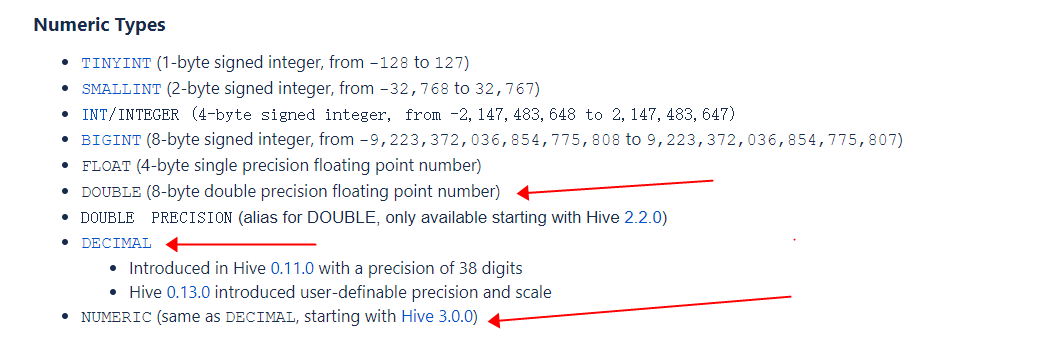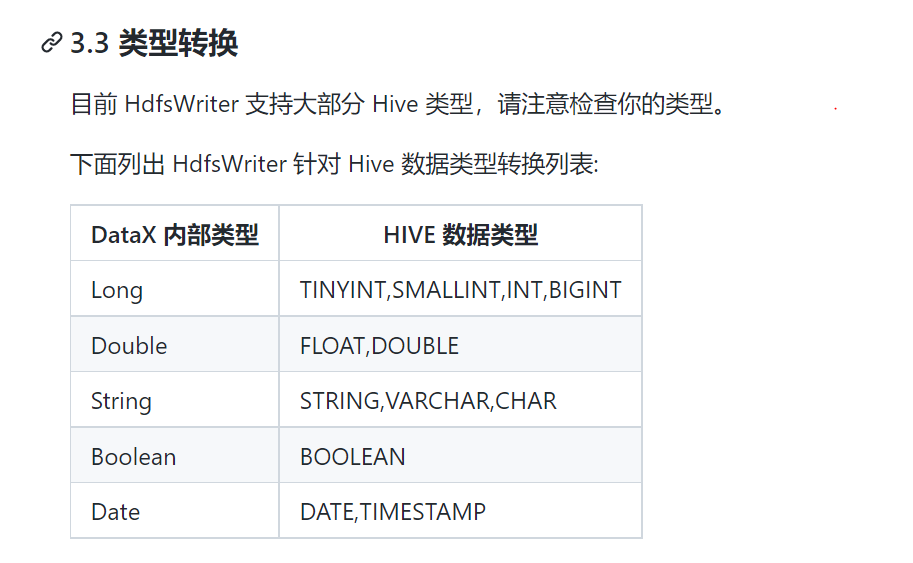IT明哥

V1

2022/10/31阅读：32主题：草原绿

# 1. JAVA 数据类型 - float/double 与 BigDecimal

• 基本数据类型 float/double;
• 基本数据类型的对应包装类 java.lang.Float/java.lang.Double;
• java.math 包下的类 java.math.BigDecimal

• Decimal data types store exact representations of numeric values, while DOUBLE data types store very close approximations of numeric values.
• Decimal types are needed for use cases in which the (very close) approximation of a DOUBLE is insufficient, such as financial applications, equality and inequality checks, and rounding operations.
• Decimal types are also needed for use cases that deal with numbers outside the DOUBLE range.

• BigDecimal 有多个构造方法，其中参数类型为 double 的构造方法的精度不能保证，其结果有一定的不可预知性，所以不推荐使用参数类型为 double 的构造方法（数值 0.1 无法准确地表示为 double数据类型）；
• BigDecimal 有多个构造方法，如果希望 BigDecimal 能够精确地表示期望的数值，那么一定要使用参数类型为 String 的构造方法，如 BigDecimal a = new BigDecimal(“0.1”) ;
• 如果不是很在乎是否完全精确地表示，并使用了 BigDecimal(double)，那么要注意 double 本身的特例，double 的规范本身定义了几个特殊的 double值 (Infinite，-Infinite，NaN)，不要把这些值传给 BigDecimal，否则会抛出异常；
• 当必须将 double 用作 BigDecimal 的数据来源时，建议先使用 Double.toString(double) 将 double 数值转换为 String，然后使用 参数类型为 String 的构造方法;
``BigDecimal a = new BigDecimal(double d); //不推荐使用参数类型为 double 的构造方法，精度不能保证；BigDecimal b= new BigDecimal(String s); //推荐使用参数类型为 String 的构造方法static BigDecimal valueOf(double d); //也可以使用 valueOf 静态方法DecimalFormat df = new DecimalFormat("#.##");// 可以使用 java.text.DecimalFormat 进行格式化，如这里保留2为小数df.setRoundingMode(RoundingMode.HALF_UP);// 可以指定具体的的舍入模式枚举类 java.math.RoundingMode，默认五舍六入，如这里指定四舍五入；``

# 2. hive 数据类型 - Double,DECIMAL,Numeric

hive 支持以下数字类数据类型，其中 FLOAT 和 DOUBLE 数据类型很早就支持了，而 Decimal 和 NUMERIC 数据类型是后续退出的：• Decimal 数据类型是在 Hive 0.11.0 (HIVE-2693) 引入的，其后在 Hive 0.13.0 (HIVE-3976) 及进行了修改重构;
• 在 Hive 0.13 之前版本，decimal 数据类型的数据精度是固定的38位；
• 在 Hive 0.13 及之后版本，声明使用 DECIMAL数据类型有三种格式：DECIMAL/DECIMAL(precision)/DECIMAL(precision, scale),用户可以使用第三种格式 DECIMAL(precision, scale) 指定 decimal 的数据精度和数据刻度（小数位数）；
• 在 Hive 0.13 及之后版本，若没有指定 DECIMAL 的精度 precision，则默认精度是10（精度的最大值是38），若没有指定小数位数 scale，则默认小数位数是 0；
• Hive 的 Decimal 数据类型底层基于 Java 的 BigDecimal，支持科学计数法和非科学计数法：
• The DECIMAL type in Hive is based on Java's BigDecimal which is used for representing immutable arbitrary precision decimal numbers in Java.
• All regular number operations (e.g. +, -, *, /) and relevant UDFs (e.g. Floor, Ceil, Round, and many more) handle decimal types.
• You can cast to/from decimal types like you would do with other numeric types.
• The persistence format of the decimal type supports both scientific and non-scientific notation. Therefore, regardless of whether your dataset contains data like 4.004E+3 (scientific notation) or 4004 (non-scientific notation) or a combination of both, DECIMAL can be used for it.
• Double 数据类型提供了数据的近似表示与近似计算，而 Decimal 数据类型提供了数据的精确表示与精确计算，且支持的数据范围更大：
• Decimal literals provide precise values and greater range for floating point numbers than the DOUBLE type.
• Decimal data types store exact representations of numeric values, while DOUBLE data types store very close approximations of numeric values；
• Decimal types are needed for use cases in which the (very close) approximation of a DOUBLE is insufficient, such as financial applications, equality and inequality checks, and rounding operations.
• They are also needed for use cases that deal with numbers outside the DOUBLE range.

SQL 语句显示转换 decimal 数据类型示例如下：

``select CAST(18446744073709001000BD AS DECIMAL(38,0));select cast(4.004E+3 AS DECIMAL(38,0));select cast(4004 AS DECIMAL(38,0));``

# 3.如何更改 datax 以支持 hive 的 DECIMAL 数据类型?

• 如上文所述，在需要对数据进行精确表示和精确运算的场景下（比如金融行业对数据准确性普遍要求较高），我们需要使用 HIVE 的 Decimal 数据类型而不是 Double 数据类型；
• 但是一些数据同步工具并不直接支持 hive 的 decimal 数据类型，此时需要基于开源版本在内部做二次开发和增强，以支持支持 hive 的 decimal 数据类型；
• 比如开源的 datax 即不支持 hive 的 decimal 数据类型：• 由于 datax 的插件化的机制，相关修改只涉及到 hdfsreader 和 hdfswriter 插件模块;
• 由于 datax 在读写 hive orc 表时，底层使用的是 hive/orc/hadoop 原生的各种 api,而这些 hive/orc/hadoop 原生的api 已经支持了对 hive Decimal 数据类型的读写操作，所以 datax 的 hdfs reader 和 hdfs writer 插件模块所需的代码修改并不多；
``-- datax 读写 hive orc 表时，底层使用的 hive/orc/hadoop 原生api部分列表如下：org.apache.hadoop.hive.ql.io.orc.OrcFile;org.apache.hadoop.hive.ql.io.orc.OrcInputFormat;org.apache.hadoop.hive.ql.io.orc.OrcOutputFormat;org.apache.hadoop.hive.ql.io.orc.OrcSerde;org.apache.hadoop.hive.ql.io.orc.Reader;org.apache.hadoop.mapred.RecordWriter;org.apache.hadoop.hive.serde2.objectinspector.ObjectInspector;-- hive读写 orc 文件数据时，底层调用的是 Core ORC API，这些 api 部分列表如下：org.apache.hadoop.hive.ql.io.orc.OrcFile;org.apache.hadoop.hive.ql.exec.vector.VectorizedRowBatch;org.apache.hadoop.hive.ql.exec.vector.ColumnVector;org.apache.hadoop.hive.ql.exec.vector.DecimalColumnVector;org.apache.hadoop.hive.ql.io.orc.Reader;org.apache.hadoop.hive.ql.io.orc.RecordReader;org.apache.hadoop.hive.ql.io.orc.Writer;org.apache.hadoop.hive.ql.io.orc.WriterImpl;org.apache.hadoop.hive.serde2.io.HiveDecimalWritable;``

## 3.1 hdfs writer 相关修改

``com.alibaba.datax.plugin.writer.hdfswriter.SupportHiveDataType;com.alibaba.datax.plugin.writer.hdfswriter.HdfsHelper#getColumnTypeInspectors;com.alibaba.datax.plugin.writer.hdfswriter.HdfsHelper#transportOneRecord(com.alibaba.datax.common.element.Record, java.util.List<com.alibaba.datax.common.util.Configuration>, com.alibaba.datax.common.plugin.TaskPluginCollector);``V1